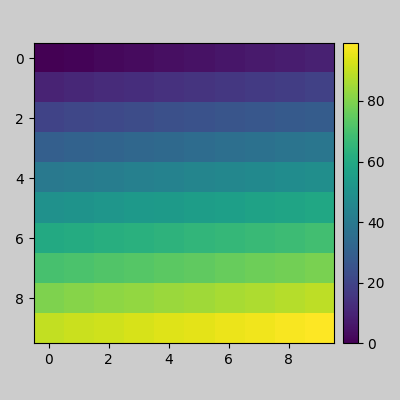# Tight Layout guide¶

How to use tight-layout to fit plots within your figure cleanly.

tight_layout automatically adjusts subplot params so that the subplot(s) fits in to the figure area. This is an experimental feature and may not work for some cases. It only checks the extents of ticklabels, axis labels, and titles.

An alternative to tight_layout is constrained_layout.

## Simple Example¶

In matplotlib, the location of axes (including subplots) are specified in normalized figure coordinates. It can happen that your axis labels or titles (or sometimes even ticklabels) go outside the figure area, and are thus clipped.

```# sphinx_gallery_thumbnail_number = 7

import matplotlib.pyplot as plt
import numpy as np

plt.rcParams['savefig.facecolor'] = "0.8"

def example_plot(ax, fontsize=12):
ax.plot([1, 2])

ax.locator_params(nbins=3)
ax.set_xlabel('x-label', fontsize=fontsize)
ax.set_ylabel('y-label', fontsize=fontsize)
ax.set_title('Title', fontsize=fontsize)

plt.close('all')
fig, ax = plt.subplots()
example_plot(ax, fontsize=24)
```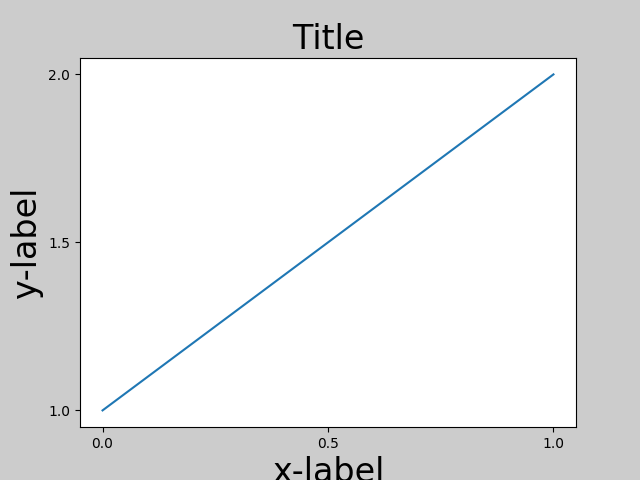To prevent this, the location of axes needs to be adjusted. For subplots, this can be done by adjusting the subplot params (Move the edge of an axes to make room for tick labels). Matplotlib v1.1 introduces a new command `tight_layout()` that does this automatically for you.

```fig, ax = plt.subplots()
example_plot(ax, fontsize=24)
plt.tight_layout()
```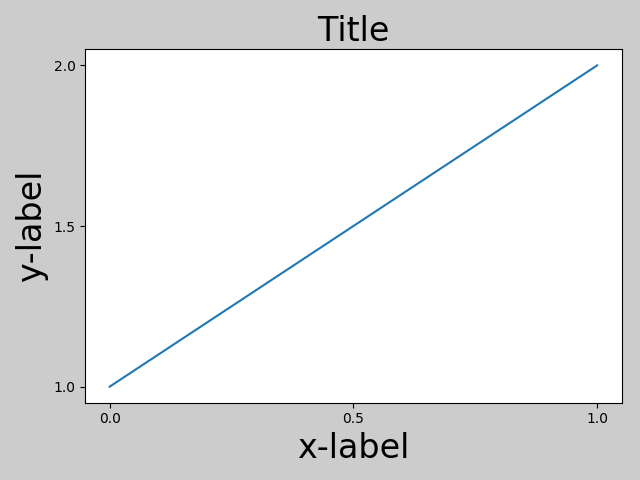Note that `matplotlib.pyplot.tight_layout()` will only adjust the subplot params when it is called. In order to perform this adjustment each time the figure is redrawn, you can call `fig.set_tight_layout(True)`, or, equivalently, set the `figure.autolayout` rcParam to `True`.

When you have multiple subplots, often you see labels of different axes overlapping each other.

```plt.close('all')

fig, ((ax1, ax2), (ax3, ax4)) = plt.subplots(nrows=2, ncols=2)
example_plot(ax1)
example_plot(ax2)
example_plot(ax3)
example_plot(ax4)
```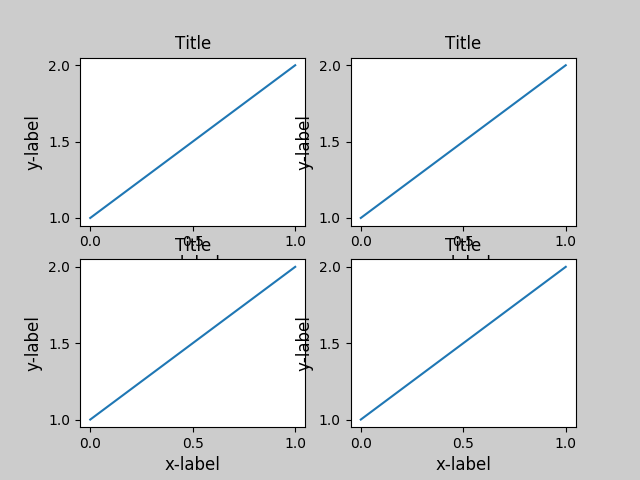`tight_layout()` will also adjust spacing between subplots to minimize the overlaps.

```fig, ((ax1, ax2), (ax3, ax4)) = plt.subplots(nrows=2, ncols=2)
example_plot(ax1)
example_plot(ax2)
example_plot(ax3)
example_plot(ax4)
plt.tight_layout()
```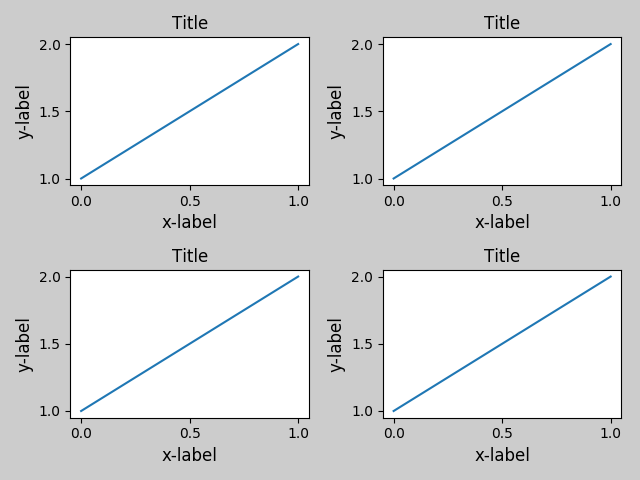`tight_layout()` can take keyword arguments of pad, w_pad and h_pad. These control the extra padding around the figure border and between subplots. The pads are specified in fraction of fontsize.

```fig, ((ax1, ax2), (ax3, ax4)) = plt.subplots(nrows=2, ncols=2)
example_plot(ax1)
example_plot(ax2)
example_plot(ax3)
example_plot(ax4)
```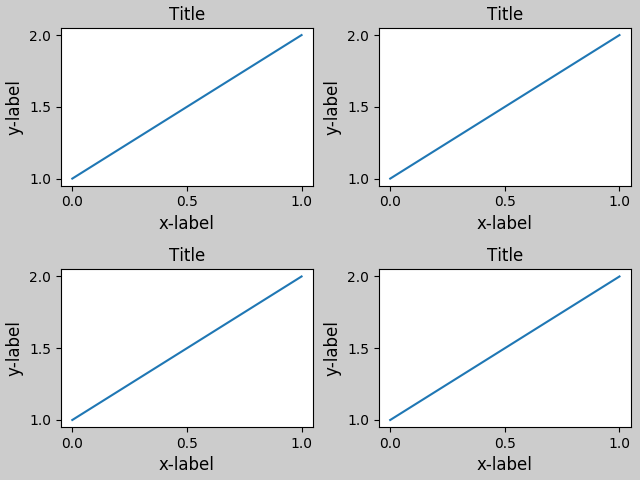`tight_layout()` will work even if the sizes of subplots are different as far as their grid specification is compatible. In the example below, ax1 and ax2 are subplots of a 2x2 grid, while ax3 is of a 1x2 grid.

```plt.close('all')
fig = plt.figure()

ax1 = plt.subplot(221)
ax2 = plt.subplot(223)
ax3 = plt.subplot(122)

example_plot(ax1)
example_plot(ax2)
example_plot(ax3)

plt.tight_layout()
```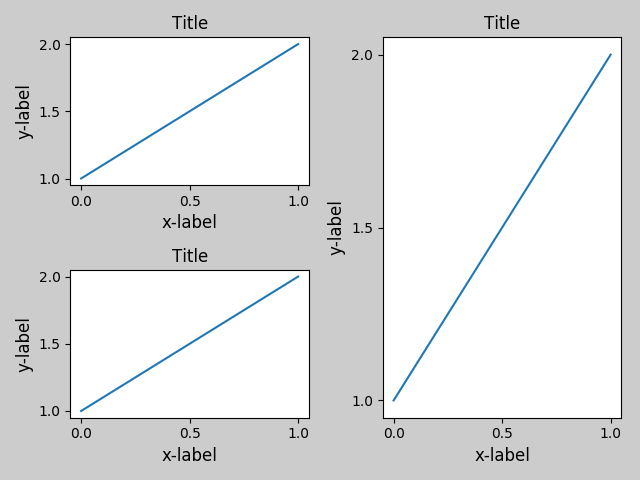It works with subplots created with `subplot2grid()`. In general, subplots created from the gridspec (Customizing Figure Layouts Using GridSpec and Other Functions) will work.

```plt.close('all')
fig = plt.figure()

ax1 = plt.subplot2grid((3, 3), (0, 0))
ax2 = plt.subplot2grid((3, 3), (0, 1), colspan=2)
ax3 = plt.subplot2grid((3, 3), (1, 0), colspan=2, rowspan=2)
ax4 = plt.subplot2grid((3, 3), (1, 2), rowspan=2)

example_plot(ax1)
example_plot(ax2)
example_plot(ax3)
example_plot(ax4)

plt.tight_layout()
```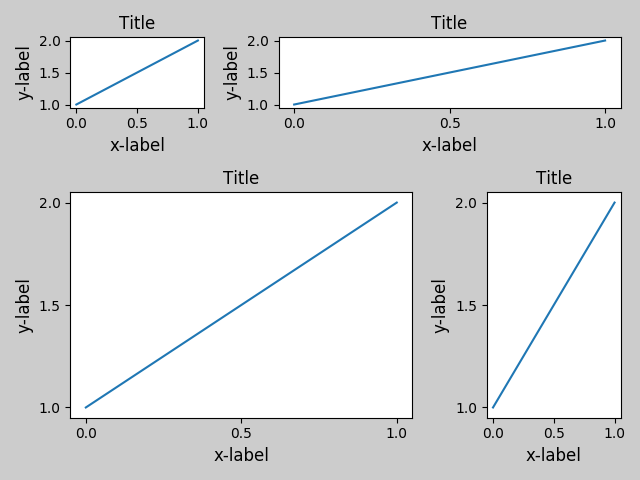Although not thoroughly tested, it seems to work for subplots with aspect != "auto" (e.g., axes with images).

```arr = np.arange(100).reshape((10, 10))

plt.close('all')
fig = plt.figure(figsize=(5, 4))

ax = plt.subplot(111)
im = ax.imshow(arr, interpolation="none")

plt.tight_layout()
```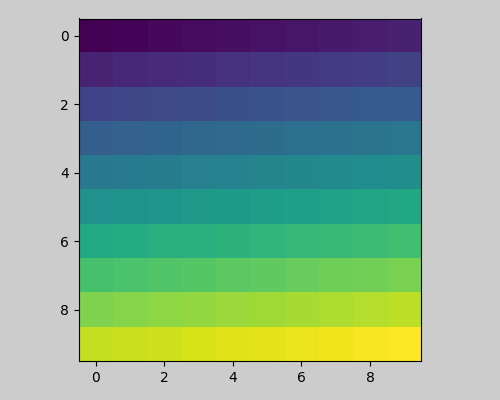## Caveats¶

• `tight_layout()` only considers ticklabels, axis labels, and titles. Thus, other artists may be clipped and also may overlap.
• It assumes that the extra space needed for ticklabels, axis labels, and titles is independent of original location of axes. This is often true, but there are rare cases where it is not.
• pad=0 clips some of the texts by a few pixels. This may be a bug or a limitation of the current algorithm and it is not clear why it happens. Meanwhile, use of pad at least larger than 0.3 is recommended.

## Use with GridSpec¶

GridSpec has its own `tight_layout()` method (the pyplot api `tight_layout()` also works).

```import matplotlib.gridspec as gridspec

plt.close('all')
fig = plt.figure()

gs1 = gridspec.GridSpec(2, 1)

example_plot(ax1)
example_plot(ax2)

gs1.tight_layout(fig)
```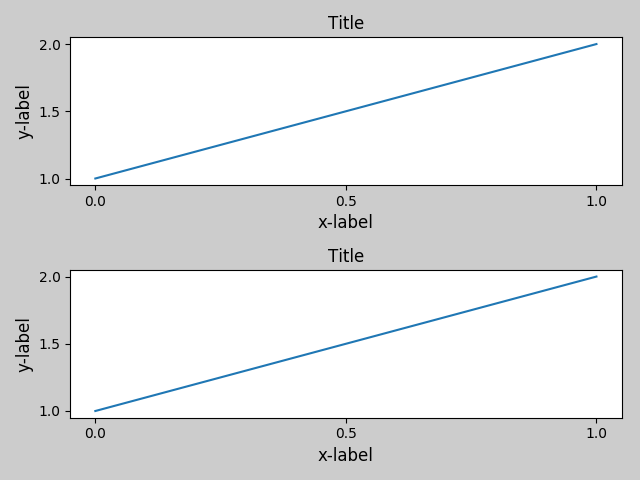You may provide an optional rect parameter, which specifies the bounding box that the subplots will be fit inside. The coordinates must be in normalized figure coordinates and the default is (0, 0, 1, 1).

```fig = plt.figure()

gs1 = gridspec.GridSpec(2, 1)

example_plot(ax1)
example_plot(ax2)

gs1.tight_layout(fig, rect=[0, 0, 0.5, 1])
```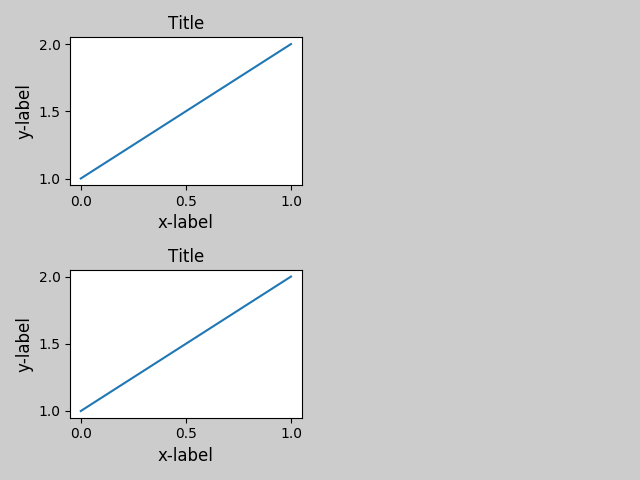For example, this can be used for a figure with multiple gridspecs.

```fig = plt.figure()

gs1 = gridspec.GridSpec(2, 1)

example_plot(ax1)
example_plot(ax2)

gs1.tight_layout(fig, rect=[0, 0, 0.5, 1])

gs2 = gridspec.GridSpec(3, 1)

for ss in gs2:
example_plot(ax)
ax.set_title("")
ax.set_xlabel("")

ax.set_xlabel("x-label", fontsize=12)

gs2.tight_layout(fig, rect=[0.5, 0, 1, 1], h_pad=0.5)

# We may try to match the top and bottom of two grids ::
top = min(gs1.top, gs2.top)
bottom = max(gs1.bottom, gs2.bottom)

gs1.update(top=top, bottom=bottom)
gs2.update(top=top, bottom=bottom)
plt.show()
```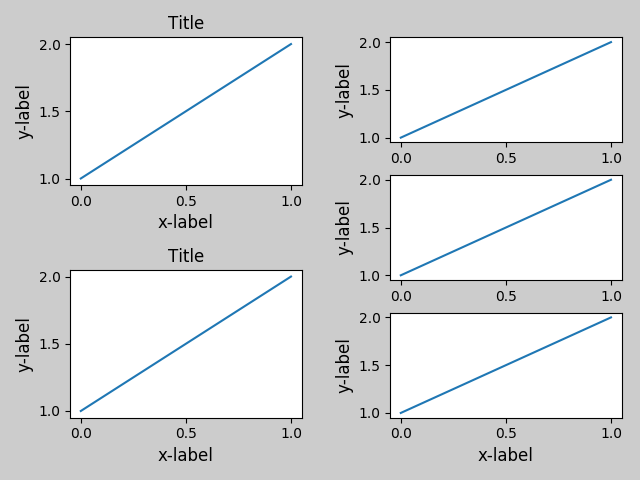While this should be mostly good enough, adjusting top and bottom may require adjustment of hspace also. To update hspace & vspace, we call `tight_layout()` again with updated rect argument. Note that the rect argument specifies the area including the ticklabels, etc. Thus, we will increase the bottom (which is 0 for the normal case) by the difference between the bottom from above and the bottom of each gridspec. Same thing for the top.

```fig = plt.gcf()

gs1 = gridspec.GridSpec(2, 1)

example_plot(ax1)
example_plot(ax2)

gs1.tight_layout(fig, rect=[0, 0, 0.5, 1])

gs2 = gridspec.GridSpec(3, 1)

for ss in gs2:
example_plot(ax)
ax.set_title("")
ax.set_xlabel("")

ax.set_xlabel("x-label", fontsize=12)

gs2.tight_layout(fig, rect=[0.5, 0, 1, 1], h_pad=0.5)

top = min(gs1.top, gs2.top)
bottom = max(gs1.bottom, gs2.bottom)

gs1.update(top=top, bottom=bottom)
gs2.update(top=top, bottom=bottom)

top = min(gs1.top, gs2.top)
bottom = max(gs1.bottom, gs2.bottom)

gs1.tight_layout(fig, rect=[None, 0 + (bottom-gs1.bottom),
0.5, 1 - (gs1.top-top)])
gs2.tight_layout(fig, rect=[0.5, 0 + (bottom-gs2.bottom),
None, 1 - (gs2.top-top)],
```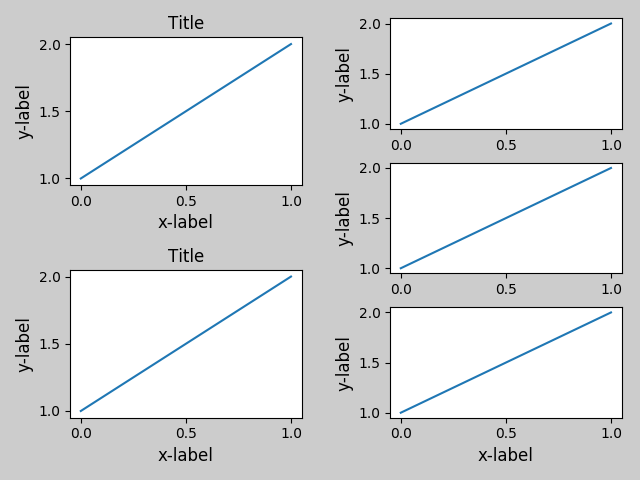## Legends and Annotations¶

Pre Matplotlih 2.2, legends and annotations were excluded from the bounding box calculations that decide the layout. Subsequently these artists were added to the calculation, but sometimes it is undesirable to include them. For instance in this case it might be good to have the axes shring a bit to make room for the legend:

```fig, ax = plt.subplots(figsize=(4, 3))
lines = ax.plot(range(10), label='A simple plot')
ax.legend(bbox_to_anchor=(0.7, 0.5), loc='center left',)
fig.tight_layout()
plt.show()
```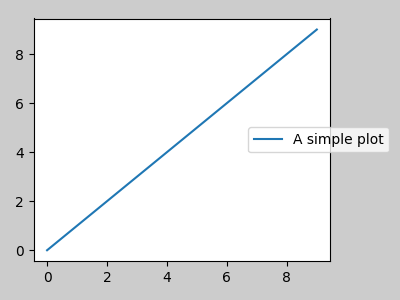However, sometimes this is not desired (quite often when using `fig.savefig('outname.png', bbox_inches='tight')`). In order to remove the legend from the bounding box calculation, we simply set its bounding `leg.set_in_layout(False)` and the legend will be ignored.

```fig, ax = plt.subplots(figsize=(4, 3))
lines = ax.plot(range(10), label='B simple plot')
leg = ax.legend(bbox_to_anchor=(0.7, 0.5), loc='center left',)
leg.set_in_layout(False)
fig.tight_layout()
plt.show()
```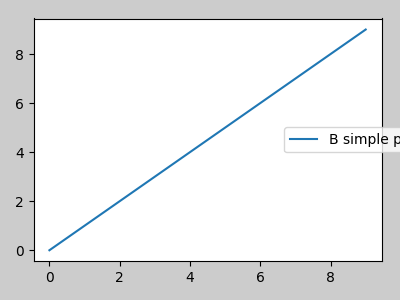## Use with AxesGrid1¶

While limited, the axes_grid1 toolkit is also supported.

```from mpl_toolkits.axes_grid1 import Grid

plt.close('all')
fig = plt.figure()
grid = Grid(fig, rect=111, nrows_ncols=(2, 2),
)

for ax in grid:
example_plot(ax)
ax.title.set_visible(False)

plt.tight_layout()
```## Colorbar¶

If you create a colorbar with the `colorbar()` command, the created colorbar is an instance of Axes, not Subplot, so tight_layout does not work. With Matplotlib v1.1, you may create a colorbar as a subplot using the gridspec.

```plt.close('all')
arr = np.arange(100).reshape((10, 10))
fig = plt.figure(figsize=(4, 4))
im = plt.imshow(arr, interpolation="none")

plt.colorbar(im, use_gridspec=True)

plt.tight_layout()
```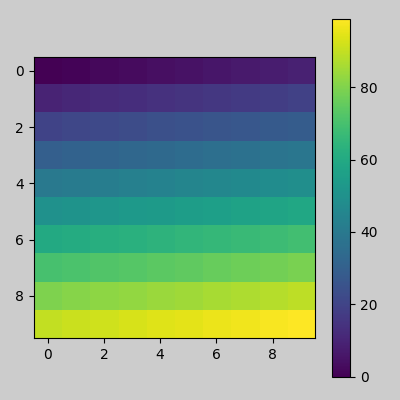Another option is to use AxesGrid1 toolkit to explicitly create an axes for colorbar.

```from mpl_toolkits.axes_grid1 import make_axes_locatable

plt.close('all')
arr = np.arange(100).reshape((10, 10))
fig = plt.figure(figsize=(4, 4))
im = plt.imshow(arr, interpolation="none")

divider = make_axes_locatable(plt.gca())